# Rhombic dodecahedron

If you divide a cube into six pyramids (the face of a cube is the base of a pyramid, the center of a cube is the vertex of a pyramid) then flip every pyramid outside the cube, you obtain a rhombic dodecahedron. It's a solid with 12 congruent faces and 14 vertices. Every face is a rhomb. There are 8 vertices adjacet to 3 edges (the vertices of the initial cube) and 6 vertices adjacent to 4 edges (the vertices of the pyramids). The rhombic dodecahedron has 24 edges.

Nick Robinson created modular origami model of the rhombic dodecahedron. Diagram of the module is avaliable on his page. This model is described in the book: David Mitchell, Mathematical Origami. Geometrical shapes by paper folding. Tarquin Publications, Norfolk, 1997, ISBN 1-899618-18-X.
On the following photo you can see a family (or kindergarten)of the rhombic dodecahedrons.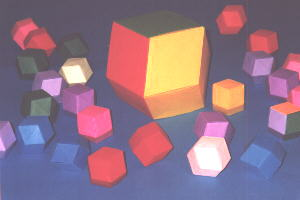Rhombic dodecahedron fills the space. It's a three-dimensional analogy of a tesselation. To prevent dodecahedrons against spilling on the table put them on the holder. I have created two holders using rhombic doecahedron module.

Here you can see both holders.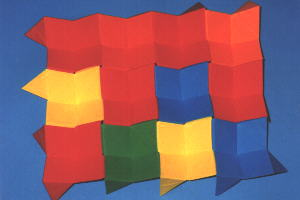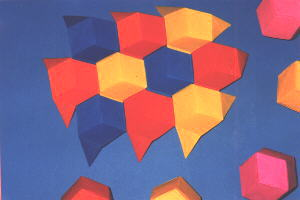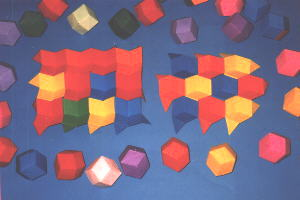And fillings of the space.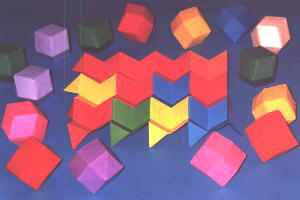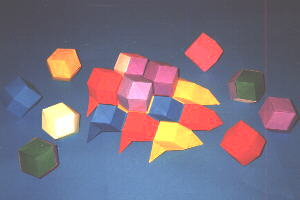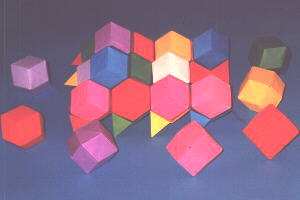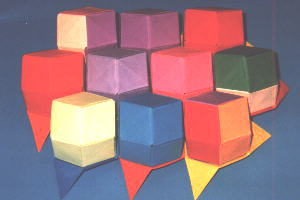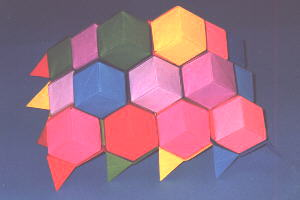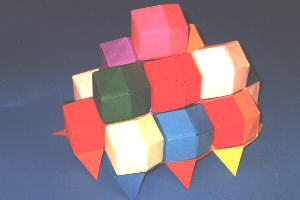Of course it's the same filing (tesselation) of the space. The only difference is the angle of the view.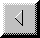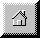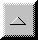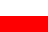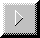Previous Home Up Map po polsku Next